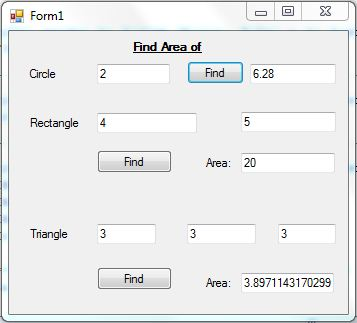VB Program for Area of Rectangle, Circle and Triangle

Tip Abstraction:
This is a VB program with description for Finding Area of Rectangle, Circle and TriangleThis is a Visual Basic program to find area of a rectangle, circle and triangle.
Ø Manually we can find the area of a rectangle by using equation:

Ø Also we can find the area of a circle by using equation:

Ø Also we can find the area of a triangle by using equation:

a = length of first side
b = length of second side
c = length of third side
s = (a + b+ c)/2

Area = sqrt(s*(s-a)*(s-b)*(s-c))
-where sqrt stands for square root

Program Code:

 Public Class Form1     Private Sub Button1_Click(ByVal sender As System.Object, ByVal e As System.EventArgs) Handles Button1.Click         TextBox2.Text = 3.14 * TextBox1.Text     End Sub     Private Sub Button2_Click(ByVal sender As System.Object, ByVal e As System.EventArgs) Handles Button2.Click         TextBox5.Text = TextBox3.Text * TextBox4.Text     End Sub     Private Sub Button3_Click(ByVal sender As System.Object, ByVal e As System.EventArgs) Handles Button3.Click         Dim a As Integer = TextBox6.Text         Dim b As Integer = TextBox7.Text         Dim c As Integer = TextBox8.Text         Dim s As Double = (a + b + c) / 2         Dim findarea As Double = Math.Sqrt(s * (s - a) * (s - b) * (s - c))         TextBox9.Text = findarea     End Sub End Class

Output:1.2.